## Example Questions

### Example Question #51 : Integers

If m and n are both even integers, which of the following must be true?

l. m2/n2 is even

ll. m2/n2 is odd

lll. m2 + n2 is divisible by four

I & III only

III only

none

II only

I only

III only

Explanation:

While I & II can be true, examples can be found that show they are not always true (for example, 22/22 is odd and 42/22 is even).

III is always true – a square even number is always divisible by four, and the distributive property tell us that adding two numbers with a common factor gives a sum that also has that factor.

### Example Question #51 : Integers

Let S be a set that consists entirely of even integers, and let T be the set that consists of each of the elements in S increased by two. Which of the following must be even?

I. the mean of T

II. the median of T

III. the range of T

I only

II only

III only

I and II only

II and III only

III only

Explanation:

S consists of all even integers. If we were to increase each of these even numbers by 2, then we would get another set of even numbers, because adding 2 to an even number yields an even number. In other words, T also consists entirely of even numbers.

In order to find the mean of T, we would need to add up all of the elements in T and then divide by however many numbers are in T. If we were to add up all of the elements of T, we would get an even number, because adding even numbers always gives another even number. However, even though the sum of the elements in T must be even, if the number of elements in T was an even number, it's possible that dividing the sum by the number of elements of T would be an odd number.

For example, let's assume T consists of the numbers 2, 4, 6, and 8. If we were to add up all of the elements of T, we would get 20. We would then divide this by the number of elements in T, which in this case is 4. The mean of T would thus be 20/4 = 5, which is an odd number. Therefore, the mean of T doesn't have to be an even number.

Next, let's analyze the median of T. Again, let's pretend that T consists of an even number of integers. In this case, we would need to find the average of the middle two numbers, which means we would add the two numbers, which gives us an even number, and then we would divide by two, which is another even number. The average of two even numbers doesn't have to be an even number, because dividing an even number by an even number can produce an odd number.

For example, let's pretend T consists of the numbers 2, 4, 6, and 8. The median of T would thus be the average of 4 and 6. The average of 4 and 6 is (4+6)/2 = 5, which is an odd number. Therefore, the median of T doesn't have to be an even number.

Finally, let's examine the range of T. The range is the difference between the smallest and the largest numbers in T, which both must be even. If we subtract an even number from another even number, we will always get an even number. Thus, the range of T must be an even number.

Of choices I, II, and III, only III must be true.

### Example Question #1 : How To Divide Even Numbers

Divide: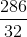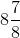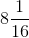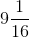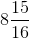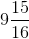Explanation:

Take a known common factor of two and rewrite the fraction.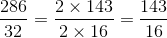Dividing the number 143 into 16, the coefficient is 8 since: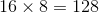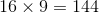There is a remainder of fifteen, which is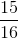.

Combining the coefficient and the remainder as a mixed fraction, this can be rewritten as: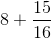The answer is:### All SAT Math Resources